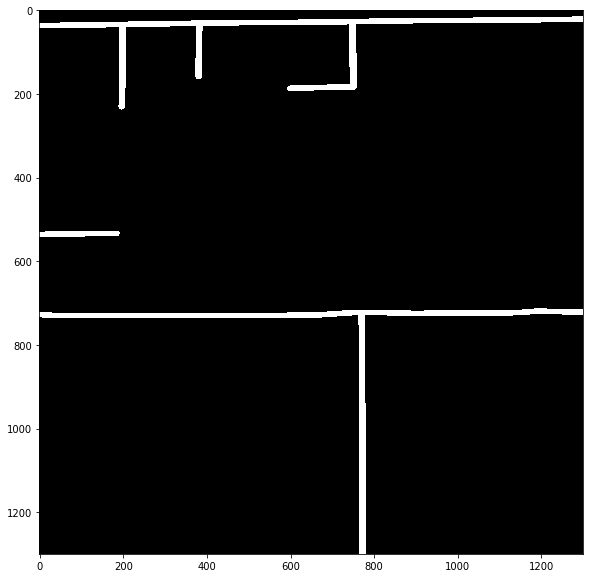# Creating training masks with the solaris python API¶

You can create training masks from geojson-formatted labels with a single solaris command. Here, we’ll go through creating masks using a sample image and geojson provided with solaris.

Solaris enables creation of four types of masks:

The first three options here can also be combined to make multi-channel training targets, as many of the SpaceNet 4 competitors did.

## Polygon footprints¶

The solaris.vector.mask.footprint_mask() function creates footprints from polygons, with 0s on the outside of the polygon and burn_value on the inside. The function’s arguments:

• df: A pandas.DataFrame or geopandas.GeoDataFrame containing polygons in one column.

• out_file: An optional argument to specify a filepath to save outputs to.

• reference_im: A georegistered image covering the same geography as df. This is optional, but if it’s not provided and you wish to convert the polygons from a geospatial CRS to pixel coordinates, you must provide affine_obj.

• geom_col: An optional argument specifying which column holds polygons in df. This defaults to "geometry", the default geometry column in GeoDataFrames.

• do_transform: Should polygons be transformed from a geospatial CRS to pixel coordinates? solaris will try to infer whether or not this is necessary, but you can force behavior by providing True or False with this argument.

• affine_obj: An affine object specifying a transform to convert polygons from a geospatial CRS to pixel coordinates. This optional (unless do_transform=True and you don’t provide reference_im), and is superceded by reference_im if that argument is also provided.

• shape: Optionally, the (X, Y) bounds of the output image. If reference_im is provided, the shape of that image supercedes this argument.

• out_type: Either int or float, the dtype of the output. Defaults to int.

• burn_value: The value that pixels falling within the polygon will be set to. Defaults to 255.

• burn_field: Optionally, a column within df that specifies the value to set for each individual polygon. This can be used if you have multiple classes.

Let’s make a mask! We’ll use sample_geotiff.tif from the sample data and geotiff_labels.geojson for polygons. First, we’ll open those two objects up so you can see what we’re working with.

:

import solaris as sol
from solaris.data import data_dir
import os
import skimage
import geopandas as gpd
from matplotlib import pyplot as plt

f, axarr = plt.subplots(figsize=(10, 10))
plt.imshow(image, cmap='gray')

:

<matplotlib.image.AxesImage at 0x7fc8ccec60b8>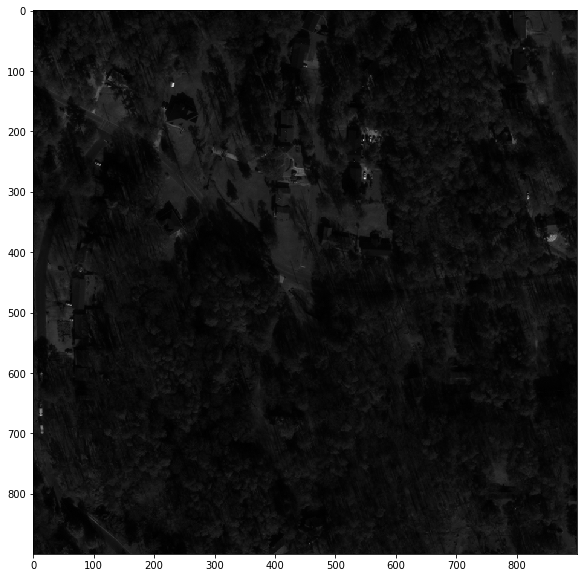This is a panchromatic image of an area in Atlanta. Can you pick out the buildings?

:

gdf = gpd.read_file(os.path.join(data_dir, 'geotiff_labels.geojson'))

:This is a convenient visualization built into Jupyter notebooks, but what are the objects it’s showing?

:

for geom in gdf.geometry[0:3]:
print(geom)

POLYGON ((733633.9175634939 3724917.327059259, 733644.0265766426 3724916.940842033, 733643.0617814267 3724892.157892002, 733632.9527424234 3724892.544111664, 733633.9175634939 3724917.327059259))
POLYGON ((733653.1326928001 3724949.324520395, 733648.855268121 3724922.585283833, 733642.8083045555 3724925.412150491, 733636.7858552651 3724926.852379398, 733638.0802132279 3724931.256500166, 733642.4909367257 3724931.19752501, 733643.0288657807 3724937.691814653, 733641.6848001335 3724942.564334524, 733641.2768538269 3724944.829464028, 733643.2673519406 3724949.61678283, 733653.1326928001 3724949.324520395))
POLYGON ((733614.6924076959 3725025.91770485, 733618.2329309537 3725025.759830723, 733618.3612589325 3725028.493043821, 733623.4073750104 3725028.260883178, 733623.2266384158 3725024.250133027, 733629.2206437828 3725023.974487836, 733628.4996830962 3725008.231178387, 733624.4670389239 3725005.347326851, 733613.7709592135 3725005.83023022, 733614.6924076959 3725025.91770485))


A bunch of Well-Known-Text (WKT)-formatted strings. Let’s convert these into something a deep learning model can use.

:

fp_mask = sol.vector.mask.footprint_mask(df=os.path.join(data_dir, 'geotiff_labels.geojson'),
reference_im=os.path.join(data_dir, 'sample_geotiff.tif'))
f, ax = plt.subplots(figsize=(10, 10))

:

<matplotlib.image.AxesImage at 0x7fc8cce33fd0>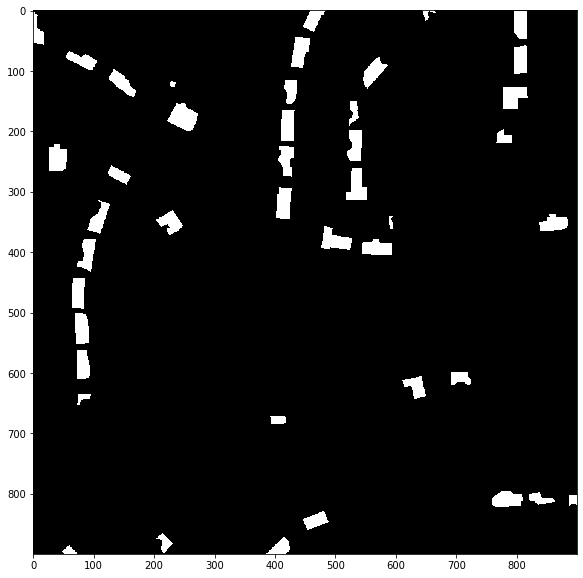This is a numpy array of shape (900, 900) with 0 at non-building pixels and 255 at building pixels.

## Polygon outlines¶

What if we want to find all of the edges of these buildings? In that case, we can use the solaris.vector.mask.boundary_mask() function. There are a few arguments to this function:

• footprint_msk: Instead of providing df as you did for footprint_mask(), for this function you can provide the footprint output. (Don’t worry, if you didn’t make one yet, you can still just provide the df argument and other footprint_mask() arguments - solaris will create it behind the scenes)

• out_file: Same as earlier.

• reference_im: Same as earlier.

• boundary_width: The width, in pixel units, of the outline around the polygon. Defaults to 3.

• boundary_type: Should the boundary be inside the polygon ("inner", the default value) or outside ("outer")?

• burn_value: Same as earlier, still defaults to 255.

You can also provide additional keyword arguments to pass to footprint_mask() if that needs to be made first.

:

b_mask = sol.vector.mask.boundary_mask(fp_mask, boundary_width=5)
f, ax = plt.subplots(figsize=(10, 10))

:

<matplotlib.image.AxesImage at 0x7fc8cc9ab6a0>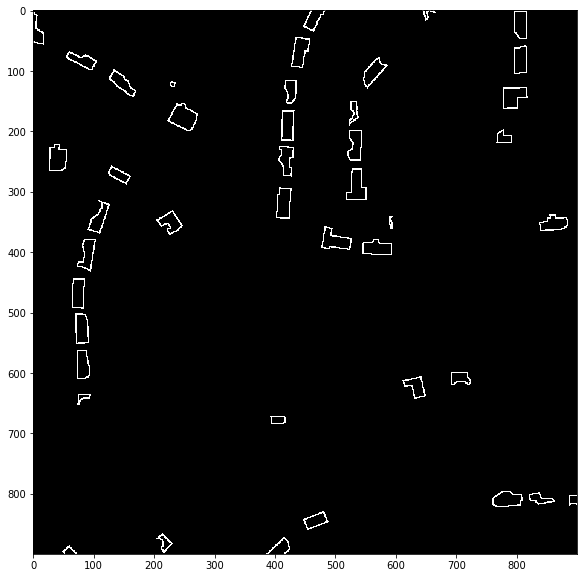Some of the SpaceNet competitors have found this type of mask useful for helping separate nearby objects.

## Polygon contact points¶

What about training a model to specifically find places where buildings are near each other? This can be helpful for the same purpose as the edges. The solaris.vector.mask.contact_mask() function creates this.

Its arguments:

• contact_spacing: An int specifying how close objects have to be for contact points to be labeled. Default value is 10.

• meters: A boolean argument indicating whether or not the contact_spacing argument is in meters. Defaults to False, in which case it’s in pixels.

This function also takes df (required), out_file, reference_im, geom_col, do_transform, affine_obj, shape, out_type, and burn_value as arguments, which have the same meanings as in solaris.vector.mask.footprint_mask().

Let’s create some contact points!

:

c_mask = sol.vector.mask.contact_mask(df=os.path.join(data_dir, 'geotiff_labels.geojson'),
reference_im=os.path.join(data_dir, 'sample_geotiff.tif'),
contact_spacing=10, meters=True)
f, ax = plt.subplots(figsize=(10, 10))

:

<matplotlib.image.AxesImage at 0x7fc8cc93f438>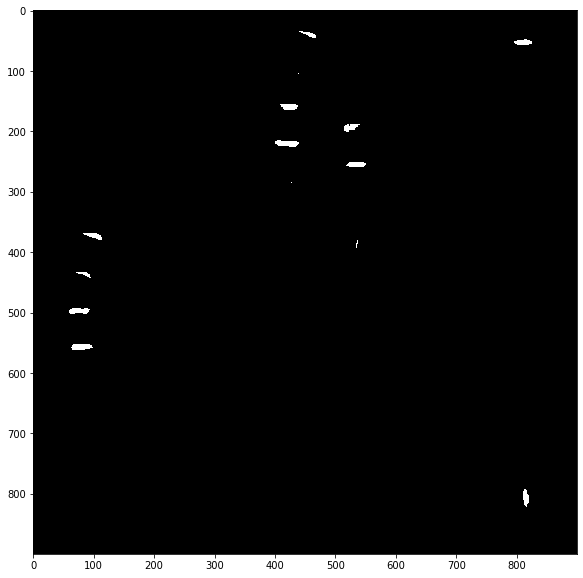Finally, let’s create a three-channel mask containing all three of these layers! The solaris.vector.mask.df_to_px_mask() function takes all of the same arguments as above, plus channels, a list specifying which of the three types of masks should be included. We’ll make one that has them all!

:

fbc_mask = sol.vector.mask.df_to_px_mask(df=os.path.join(data_dir, 'geotiff_labels.geojson'),
channels=['footprint', 'boundary', 'contact'],
reference_im=os.path.join(data_dir, 'sample_geotiff.tif'),
boundary_width=5, contact_spacing=10, meters=True)
f, ax = plt.subplots(figsize=(10, 10))

:

<matplotlib.image.AxesImage at 0x7fc8cc81d6a0>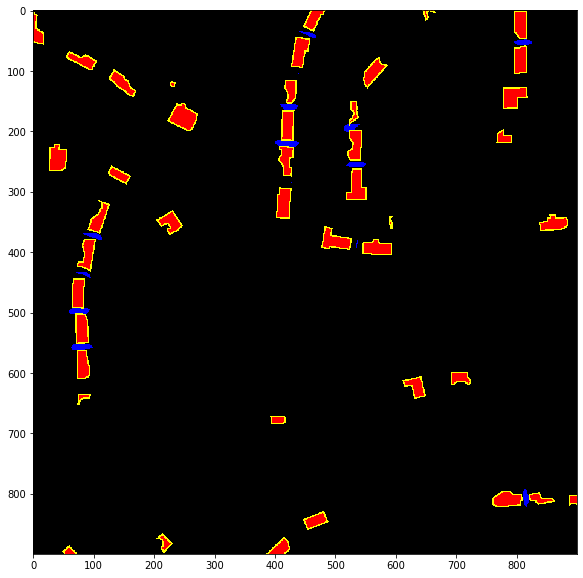Finally, we can use linestrings to create a road network. Here we’re going to use a different sample input image and geojson alongside the solaris.vector.mask.road_mask(). This function takes many of the same arguments as the earlier functions: df (required), meters, out_file, reference_im, geom_col, do_transform, affine_obj, shape, out_type, burn_value, and burn_field.

This function also takes width, which specifies the width of the road network (in pixels unless meters=True).

:

road_mask = sol.vector.mask.road_mask(os.path.join(data_dir, 'sample_roads_for_masking.geojson'),

:

<matplotlib.image.AxesImage at 0x7fc8cc8a14a8>